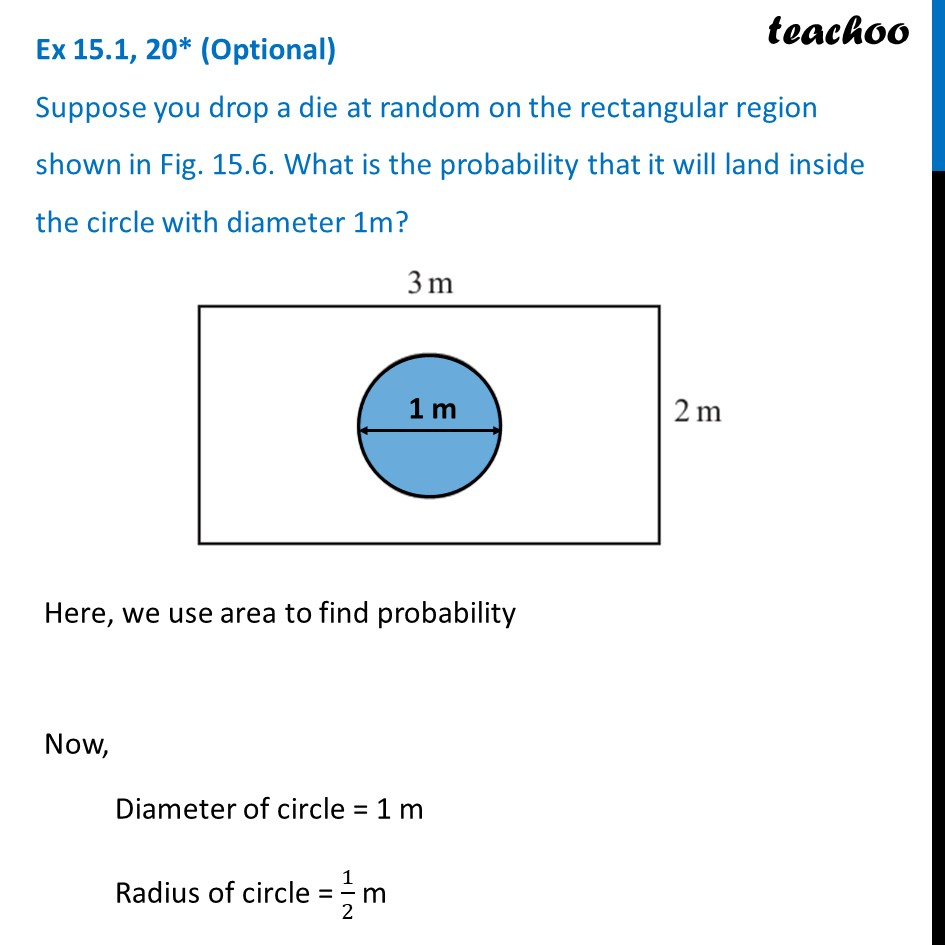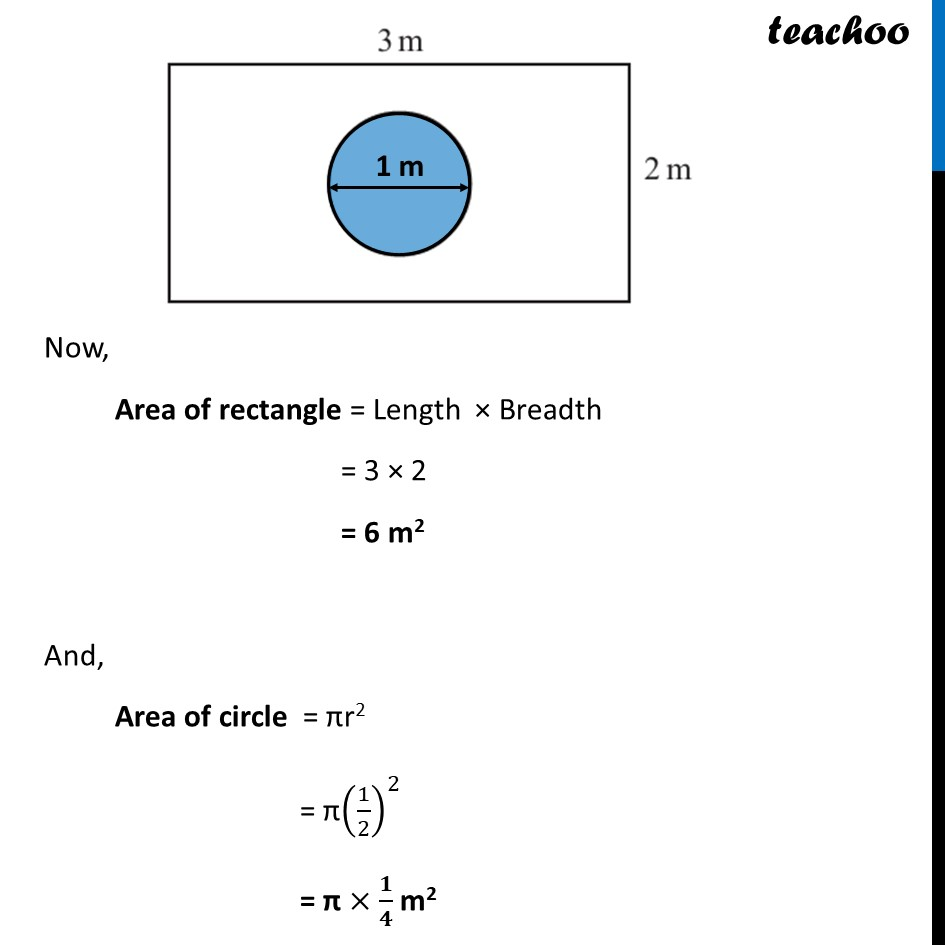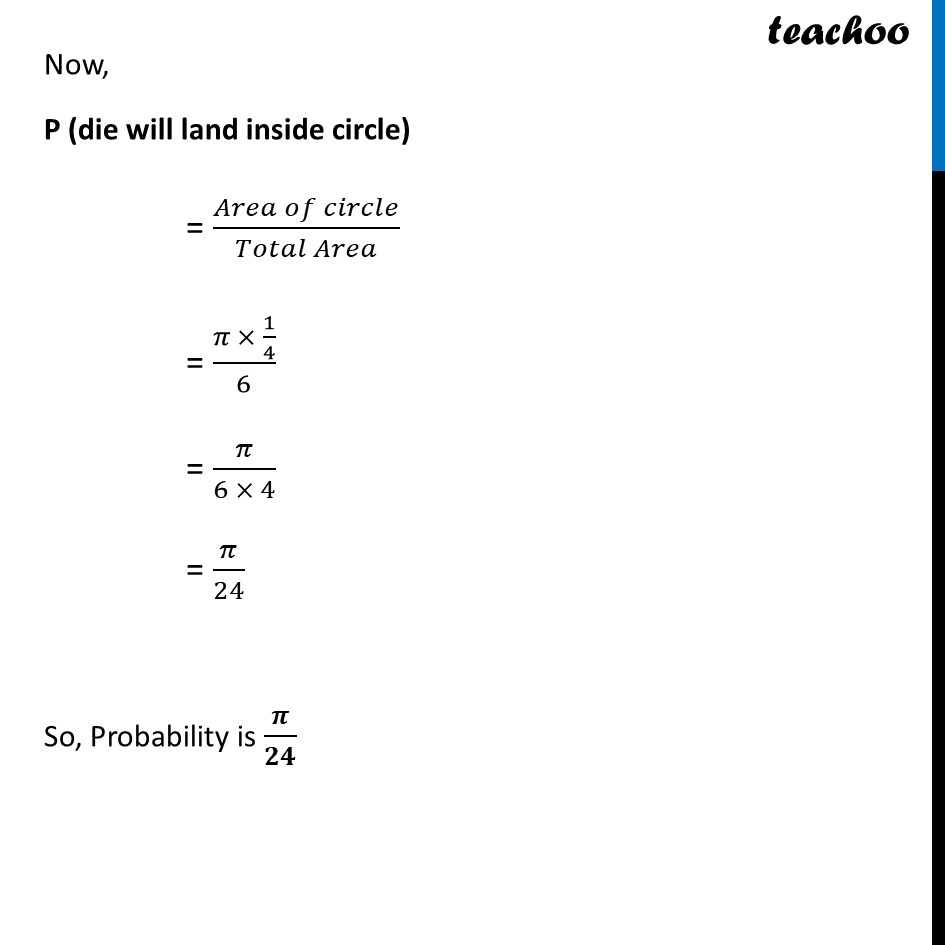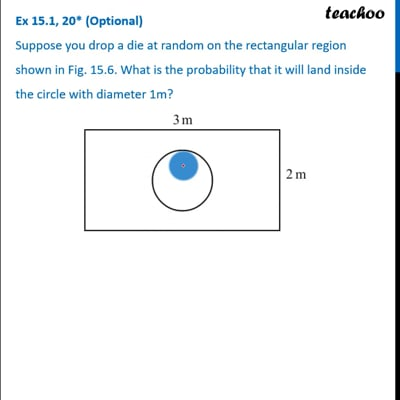Ex 15.1

Chapter 15 Class 10 Probability
Serial order wiseThis video is only available for Teachoo black users

Introducing your new favourite teacher - Teachoo Black, at only ₹83 per month

### Transcript

Ex 15.1, 20* (Optional) Suppose you drop a die at random on the rectangular region shown in Fig. 15.6. What is the probability that it will land inside the circle with diameter 1m? Here, we use area to find probability Now, Diameter of circle = 1 m Radius of circle = 1/2 m Now, Area of rectangle = Length × Breadth = 3 × 2 = 6 m2 And, Area of circle = πr2 = π(1/2)^2 = π ×𝟏/𝟒 m2 Now, P (die will land inside circle) = (𝐴𝑟𝑒𝑎 𝑜𝑓 𝑐𝑖𝑟𝑐𝑙𝑒)/(𝑇𝑜𝑡𝑎𝑙 𝐴𝑟𝑒𝑎) = (𝜋 × 1/4)/6 = 𝜋/(6 × 4) = 𝜋/24 So, Probability is 𝝅/𝟐𝟒